Download Presentation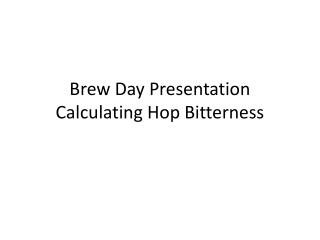Brew Day Presentation Calculating Hop Bitterness

# Brew Day Presentation Calculating Hop Bitterness - PowerPoint PPT Presentation

Brew Day Presentation Calculating Hop Bitterness. Measuring Hop Bitterness . Homebrew Bittering Units (HBUs) HBUs= Hop Wt. in (ounces)*Alpha Acid Percent For example, if you use 2.5 ounces of Cascade Hops with an alpha acid of 6.5% then the HBUs= 2.5*6.5 = 16.25 homebrew units .I am the owner, or an agent authorized to act on behalf of the owner, of the copyrighted work described.
Download Presentation## Brew Day Presentation Calculating Hop Bitterness

An Image/Link below is provided (as is) to download presentation

Download Policy: Content on the Website is provided to you AS IS for your information and personal use and may not be sold / licensed / shared on other websites without getting consent from its author.While downloading, if for some reason you are not able to download a presentation, the publisher may have deleted the file from their server.

- - - - - - - - - - - - - - - - - - - - - - - - - - E N D - - - - - - - - - - - - - - - - - - - - - - - - - -
Presentation Transcript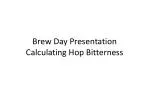### Brew Day PresentationCalculating Hop Bitterness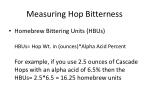Measuring Hop Bitterness
• Homebrew Bittering Units (HBUs)HBUs= Hop Wt. in (ounces)*Alpha Acid PercentFor example, if you use 2.5 ounces of Cascade Hops with an alpha acid of 6.5% then the HBUs= 2.5*6.5 = 16.25 homebrew units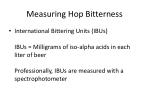Measuring Hop Bitterness
• International Bittering Units (IBUs)IBUs = Milligrams of iso-alpha acids in each liter of beerProfessionally, IBUs are measured with a spectrophotometer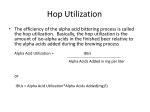Hop Utilization
• The efficiency of the alpha acid bittering process is called the hop utilization. Basically, the hop utilization is the amount of iso-alpha acids in the finished beer relative to the alpha acids added during the brewing processAlpha Acid Utilization = IBUs Alpha Acids Added in mg per liter

orIBUs = Alpha Acid Utilization*Alpha Acids Added(mg/l)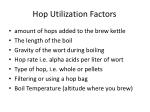Hop Utilization Factors
• amount of hops added to the brew kettle
• The length of the boil
• Gravity of the wort during boiling
• Hop rate i.e. alpha acids per liter of wort
• Type of hop, i.e. whole or pellets
• Filtering or using a hop bag
• Boil Temperature (altitude where you brew)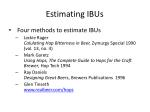Estimating IBUs
• Four methods to estimate IBUs
• Jackie RagerCalulating Hop Bitterness in Beer, Zymurgy Special 1990 (vol. 13, no. 4)
• Mark GaretzUsing Hops, The Complete Guide to Hops for the Craft Brewer, Hop Tech 1994
• Ray DanielsDesigning Great Beers, Brewers Publications 1996
• Glen Tinsethwww.realbeer.com/hops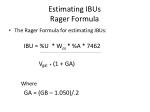Estimating IBUsRager Formula
• The Rager Formula for estimating IBUs: IBU = %U * Woz * %A * 7462

Vgal * (1 + GA)

Where

GA = (GB – 1.050)/.2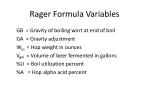Rager Formula Variables

GB = Gravity of boiling wort at end of boil

GA = Gravity adjustment

Woz = Hop weight in ounces

Vgal = Volume of beer fermented in gallons

%U = Boil utilization percent

%A = Hop alpha acid percent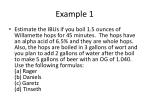Example 1
• Estimate the IBUs if you boil 1.5 ounces of Willamette hops for 45 minutes. The hops have an alpha acid of 6.5% and they are whole hops. Also, the hops are boiled in 3 gallons of wort and you plan to add 2 gallons of water after the boil to make 5 gallons of beer with an OG of 1.040. Use the following formulas:(a) Rager(b) Daniels(c) Garetz(d) Tinseth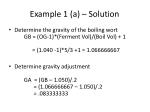Example 1 (a) – Solution
• Determine the gravity of the boiling wort GB = (OG-1)*(Ferment Vol)/(Boil Vol) + 1 = (1.040 -1)*5/3 +1 = 1.066666667
• Determine gravity adjustment GA = (GB – 1.050)/.2 = (1.066666667 – 1.050)/.2 = .083333333Example 1(a) – Solution con’t
• Determine the IBUs IBUs = .269 * 1.5 * .065 * 7462 5 * (1 + .08333333) = 36.13Estimating IBUsDaniels Formula
• The formula from Ray Daniels to estimate IBUs is similar to Rager except for the conversion factor and the %U values: IBU = %U * Woz* %A * 7489

Vgal * (1 + GA)

Where

GA = (GB – 1.050)/.2Example 1 (b) – Solution
• Determine the gravity of the boiling wort GB = (OG-1)*(Ferment Vol)/(Boil Vol) + 1 = (1.040 -1)*5/3 +1 = 1.066666667
• Determine gravity adjustment GA = (GB – 1.050)/.2 = (1.066666667 – 1.050)/.2 = .083333333Example 1 (b) – Solution con’t
• Determine the IBUs IBUs = .22 * 1.5 * .065 * 7489 5 * (1 + .083333333) = 29.66Estimating IBUsGaretz Formula
• Mark Garetz’s formula for estimating IBUs is also similar to Rager, except Garetz adds a temperature factor and a hopping rate factor and the %U values are different.The Garetz Formula for estimating IBUs:IBU = %U * Woz* %A * 7490

Vgal * (1 + GA) * TF * HFEstimating IBUsGaretz Formula

Where

GA = (GB – 1.050)/.2

TF = ((Elevation in feet/550)*0.02) + 1CF = (Ferment Vol)/(Boil Vol)HF = (CF*IBU/260) + 1Estimating IBUsTinseth Formula
• Glenn Tinseth’s formula for calculating IBUs uses a Bigness factor and a Boil time factor to determine the overall utilization. Here is the formula:IBU = %U * Woz * %A * 7490

VgalEstimating IBUsTinseth Formula

Where %U represents total utilization and is defined as:

%U= Bigness factor * Boil time factor

Bigness factor = 1.65*.000125^(average wort boil gravity-1)

Boil Time factor = 1 – e^(-.04*time in mins) 4.15